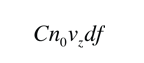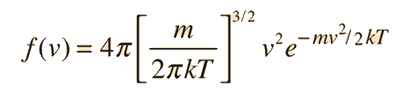# Relation of particle velocity to height

Molecules which are higher in the atmosphere are there ultimately because they had a higher kinetic energy to convert into gravitational potential energy on some time scale. (Never mind the fact of untold billions of collisions on their way to that height!) On a very short time scale between collisions, some will rise incrementally higher than others from their starting points. It is that incremental height gain we consider here.)

If we have a population of particles which have energy sufficient to reach height z:then a population with a little higher velocity can get a little higherThese energy considerations must account for the difference in population with height we observe in the barometric formula.
 Back to Discussion of Maxwell speed distribution development Forward to Relate particle flux to the velocity distribution.
Index

Gas law concepts

Kinetic theory concepts

 HyperPhysics***** Heat and Thermodynamics R Nave
Go Back

# Relation of particle flux to velocity distribution

The number of particles traveling from z to z + dz per unit time may be related to the velocity distribution f(v). The number of particles per unit volume available for the trip isFor a given velocity vz, the number which would make it is given by multiplying the number per unit volume times the volume swept outThe general expression for the change in population (put in differential form) is:where df is the differential of the velocity distribution f. So the flux is seen to be proportional to the upward velocity.

 Back to Discussion of Maxwell speed distribution development Forward to Relate velocity distribution to the barometric formula
Index

Kinetic theory concepts

 HyperPhysics***** Heat and Thermodynamics R Nave
Go Back

# Relate the velocity distribution to the barometric formula

The difference in particle population with height from the barometric formula iswhich must be driven by the available energy for the distribution. The differential change with height isand it can be related to the net particle fluxFor these small excursions in z, which are chosen small enough that no collisions occur, the height z attained can be related to the particle kinetic energy:The constant C is used to lump together the scaling factors.

 Back to Discussion of Maxwell speed distribution development Forward to Develop and normalize the velocity distribution function.
Index

Kinetic theory concepts

 HyperPhysics***** Heat and Thermodynamics R Nave
Go Back

# The form of the velocity distribution

The form of the velocity distribution in one dimension can be taken from the Boltzmann distribution in terms of particle kinetic energy. Here we are trying to argue the plausibility of that relationship is by specific correlation with the barometric formula, from which we have obtained:.

Since the integral over all values of velocity must be equal to 1, the expression can be normalized toShow

This is the final step in an exercise to show that one can arrive at the Boltzmann distribution by starting with two experimental observations: the equipartition of energy and the barometric formula. It is in no sense a derivation of the Boltzmann distribution, but one more exercise to show the self-consistency of a major relationship in nature upon which we depend heavily in the study of thermodynamics and kinetic theory.

 Discussion of Maxwell speed distribution development
Index

Kinetic theory concepts

 HyperPhysics***** Heat and Thermodynamics R Nave
Go Back

# The 3-Dimensional Velocity Distribution

The form of the velocity distribution in one dimension can be taken from the Boltzmann distribution in terms of particle kinetic energy. One way to argue the plausibility of that relationship is by specific correlation with the barometric formula, from which we have obtained:which is a Boltzmann distribution for which the probability increases exponentially with decreasing energy. There is no evidence of a peak in probability at some finite value. This is a gaussian distribution around zero velocity, since with purely random motion, the average vector particle velocity is zero. Why, then, is the Maxwell speed distributionskewed by the velocity squared factor toward higher speeds? The qualitative answer is that there are more ways to achieve the higher speeds when all directions in space are considered. The integration of the velocity distribution function over a range of velocities is essentially a "volume" integral where the velocity vector represents the "radius" of the volume. When you sum over all the probabilities for a given increment dv, it encloses more "volume" if that increment is at higher v.
 Discussion of Maxwell speed distribution development Maxwell speed distribution directly from Boltzmann
Index

Kinetic theory concepts

 HyperPhysics***** Heat and Thermodynamics R Nave
Go Back